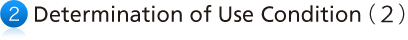##(2) Calculation method of coefficient of revolution velocity

(a) Calculate the circumferential velocity of joint outer diameter.T₁＝transmission torque(N・m)
N＝use revolution(min⁻¹)
KW＝prime mover capacity

(b)Based upon the figure V1 (circumferential velocity of outer diameter) from the above　formula, obtain the coefficient of revolution velocity using the following graph